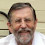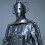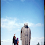### The Event Horizon

Event Horizon was a somewhat dodgy sci-fi-horror movie that came out in the late 1990s. As the title suggests, associated with an Event Horizon is "Infinite Space, Infinite Terror". Luckily, the Event Horizon in the film as a black hole Event Horizon, and I'll leave discussing those to another time. Today, we'll try and understand the Cosmological Event Horizons.

To explain these, I am shamelessly going to use the (still) excellent cosmological figures produced by Tamara Davis. OK, let's start with this one.
To understand what this picture is telling us, we need to remember a few things. Our universe has three spatial dimensions, and any spatial point can be labelled with three numbers. In a Cartesian coordinate system, these are (x,y,z). As we are dealing with relativity, we are dealing with not only space, but space-time, and every point in the universe is labelled by 4 numbers, the three spatial coordinates and the time, t. So, every point is labelled as (t,x,y,z), and these points are called an Event.

The picture above is the evolution of our universe, the ΛCDM cosmological model, and has distance on the x-axis and time on the y-axis. The universe began at a finite time in the past (the Big Bang) and shows us (the vertical line in the centre) and other objects in the universe. At the Big Bang, distances between us and any other object is zero, and as the universe expands, objects move away from us.

The purple is the Hubble Sphere that we discussed last time. The question is, what are those other lines on there - the event horizon and the particle horizon? To answer this, we need to do a bit of mathemagic.

We need to note a couple of things. Firstly, the first picture is not the complete story, as we know that, if our current cosmology is correct, then while it was born a finite time in the past, it's going to last for ever. So really the first figure goes on for ever also.  But we can fix that and come to it in a moment.

The x-axis actually shows "physical distance". Now, the proper distance is the multiplication of the Scale Factor (which depends on time and changes as the universe evolves) and what's known as the Comoving Coordinates (which, for any individual galaxy, are fixed values).

The Scale Factor's evolution depends upon what the universe is made of, and here's a few some people made earlier
The things to see here that now is at t=0, and the scale factor was smaller in the past (things were closer together) and at some time way-back-when the scale factor was zero (the Big Bang). If we divide out the Scale Factor (so each galaxy has only its fixed comoving coordinates) we get the following picture

What are we going to do about the time axis? There is some mathemagic we can do with that also. But what, as we know that the eventual age of the universe stretches off to infinity. I'm not going to go through the gory mathematical details, but we are going to switch from the normal cosmological time to what is known as Conformal Time. For our particular universe, the cool think is that the infinite age of the universe is mapped onto a fine conformal time.

This can give you a bit of a headache, but we know lots of functions that can map the infinite onto the finite (and mathematicians, don't complain about the terminology here :), such as tanh(x). So, when we look at our universe in terms of comoving coordinate and conformal time, we get the following
Remember, in terms of time, this is the entire history of the Universe, from Big Bang to infinite future, all on one piece of paper.

Now, the cool thing, the really cool think with these coordinates is that light rays travel at 45 degrees, not at the crazy curves we see above, and we see that our event horizon is made of such straight lines, meeting at where we head into the finite conformal infinity.

So, where does this get us? Well, the event horizon forms a triangle, separating events which are inside the triangle from those outside. Remembering that light rays travel at 45 degrees on this picture, and sit down with a pencil and a ruler, what you can see what this separation of events means.

If we pick an event within the triangle (remember, this is just a dot on this page) we can draw a light ray (travelling at 45 degrees) which hits us at the origin, somewhere between the Big Bang and the infinite future.

But if we choose an event outside of the event horizon and draw another light ray heading towards us, we will see that it will not be able to cross our path between the Big Bang and infinite future.

So, this means that the event horizon separates events from those that can ever send us a signal (i.e. we can see at some point in our history) from those that can't. The proper way of saying this is that the event horizon separates events into those that can have Causal Contact with us, from those that cannot.

This might seem weird, as if you think of a distant galaxy sending our light to us from a finite distance away, then, giving the fact that the universe will be infinitely old, we must receive the light at some point? But no, because the photon is battling the expansion of the universe, and may not win and we may never see it.

There is a flip side to this and if we take the above figure and extend the red light (our light cone) to the top of the picture, we can see something quite interesting. If we set off in a standard rocket, we can never travel faster than light, and so will be always within the future red triangle. What this means is that even though we have an infinite amount of time left to play in the universe, we can only explore this finite patch (as thing we are trying to get to are being pulled away from us by the cosmic expansion).

In fact, the longer we leave it, the less and less volume there is to explore! So we'd better head off right away if we are going to see anything!

1.Geraint,

It is truly fascinating to see the entire Universe - past, present and future - reduced to three 'simple' graphs. I'm grappling with some of the concepts but I think I may be able to fully understand them, maybe after a few reads. I certainly understand a lot more now than I did before I read your two related blogs.

I am still not clear of the fundamental difference between the two definitions of the Hubble Sphere and the Event Horizon.

* The 'Hubble Sphere' denotes the distance at which the perceived expansion of the Universe matches the speed of light, from our perspective. Beyond it, no signal can reach us. I get that.

* The 'Event Horizon' is the distance beyond which there can be no causal effect on us. I get that.

To me the two definitions appear identical, yet there is obviously a subtle difference in definition which I have missed. What is special about that yellow shaded area? How is it that an event can have a causal effect without any signal being able to reach us?

Finally, would I be right in saying that, on the first Gyr/Glyr graph, if you raised the blue "now" bar further up the page, billions of years into the future, that the red light cone would tend towards taking up the space and shape of the event horizon?

Thanks

Roger Powell

2.Hi - Great questions.

OK - First part - What's the difference between the Hubble Sphere and the Event Horizon?

Objects within the Hubble Sphere *now* are moving away from us less than the speed of light. Those outside are moving faster than the speed of light. Now, remember that these velocities are due to the expansion of the universe.

So, you might think that objects outside the Hubble Sphere now would never be observable. But remember that the expansion of the universe can speed up and slow down (it depends on what the energy mix in the universe is) and so if it slows, an object outside of the Hubble Sphere *now* might end up inside the Hubble Sphere in the future, and so be visible in the future.

Events outside the event horizon will never be visible to us.

As for your second question, yes, you are right (and you can see this more clearly in the final picture). Basically, the event horizon can be thought of as the ultimate light cone in the universe.

I should note, however, that not every universe has an event horizon. If the conformal time does not converge to a finite value as the age of the universe goes to infinity, then we can't draw an ultimate light cone, and we could explore the entire universe.

Hope this make sense.

3.Thanks Geraint, that makes it somewhat clearer but I have to ask a follow up question:
How would you define an event (say on graph 1) that lies outside our Hubble Sphere but which remains within our light cone, i.e the small parts of the yellow bananas that lie inside the red cone?
How can our light cone contain space-time events which are expanding away from us faster than light speed?
Are such events observable or not?
Is this something to do with the changing value of the Hubble Constant?

4.OK - Firstly, the distance to the Hubble sphere is D = c/H where c is the speed of light and H is Hubble's constant (you actually have a slightly more complex relation for non-spatially flat universe).

Of course, Hubble's constant is not a constant, but changes with time, and so the distance to the Hubble Sphere changes with time (and you can see this in the figures above).

So, how fast an object is moving away depends upon Hubble's constant, and objects can initially be moving faster than light, but later be slower then light.

Can an event in the yellow banana be seen? Well, this is easy to see. The events we see right now are the ones on the past light cone (the red line) and if you consider an event in the yellow on the red line, the red line (the photons) are initially moving outwards, but eventually turns around and heads back to the observer, so yes, they are observable.

How's that?

1."Firstly, the distance to the Hubble sphere is D = c/H where c is the speed of light and H is Hubble's constant (you actually have a slightly more complex relation for non-spatially flat universe)."

Actually, if D is the proper distance (which is the case in the velocity-distance relation v=HD), then this is the case in all cosmological models, spatially flat or not.

5.A fair bit clearer, thanks Geraint.

The concept of light "turning around" is an interesting one to ponder and it makes that entire banana zone very intriguing.

It seems to me that the furthest extent of the light cone (in the X direction, or proper distance) coincides with it's intersection of the Hubble sphere and I assume this is the turning point where light which was previously receding due to expansion begins to approach.

I guess it all comes back to my earlier question and your response that "the event horizon can be thought of as the ultimate light cone in the universe."

Thanks very much for your explanations,

Roger

1.Your right it's called the Loop theory when my book is finished the world will know about<[space/time/vacuum/continuum]>©light dose bend going through the black hole it dose seem to bend around stars but only reflects to give them a prism effect.

2.Your right it's called the Loop theory when my book is finished the world will know about<[space/time/vacuum/continuum]>©light dose bend going through the black hole it dose seem to bend around stars but only reflects to give them a prism effect.

6.An easier way to think of this is the following: Think of a flash of light at the big bang, at our comoving location. The surface of this expanding sphere of light is the particle horizon.

The event horizon is the same thing in reverse. Think of emitting a flash of light now: the farthest distance it will reach is the event horizon.

In both cases, of course, due to symmetry we can think of light travelling toward us rather than away from us. At a given time, the particle horizon is at the farthest distance from which light could have reached us. The event horizon is the distance such that light emitted there now will reach us in the infinite future or at the big crunch.

The universe can have a particle horizon, an event horizon, both or neither. These can grow, shrink, or remain constant in time. In general, these have nothing to do with the Hubble sphere, though in some special cases there can be some degeneracy (for example, in the de Sitter model the Hubble sphere is the event horizon).

One can think of either horizon as a surface in space at a given time or a surface in space-time. Often, the former is emphasized in the discussion of the particle horizon and the latter in the case of the event horizon (including in Rindler's classic paper, though he does mention all possibilities); this creates some confusion and obscures the essential symmetry. Case in point: Do universes which end in a big crunch have an event horizon? For space-time yes, since time ends; for space usually (always?) not since all distances are eventually reached.

As almost always, if you want to understand basic concepts in cosmology, read Harrison's textbook:

Author: Edward R. Harrison
Title: Cosmology: The Science of the Universe (2nd Edition)
Publisher: Cambridge University Press
Year: 2000/2001
ISBN: ISBN 0-521-66148-X

7.I believe, conversely that light cones are local event horizons. Outside of the light cone you would be elsewhere in space and time. Thus the Higgs field prevents matter from exceeding the speed of light and escaping the universe.

8.I believe also, that classical and quantum physics merge when the speed of light is achieved by anything. This is consequence of the time dimension locally being zero. Thus the object loses all causality and becomes a two dimensional possibility cloud or wave on the light cone, just like light.